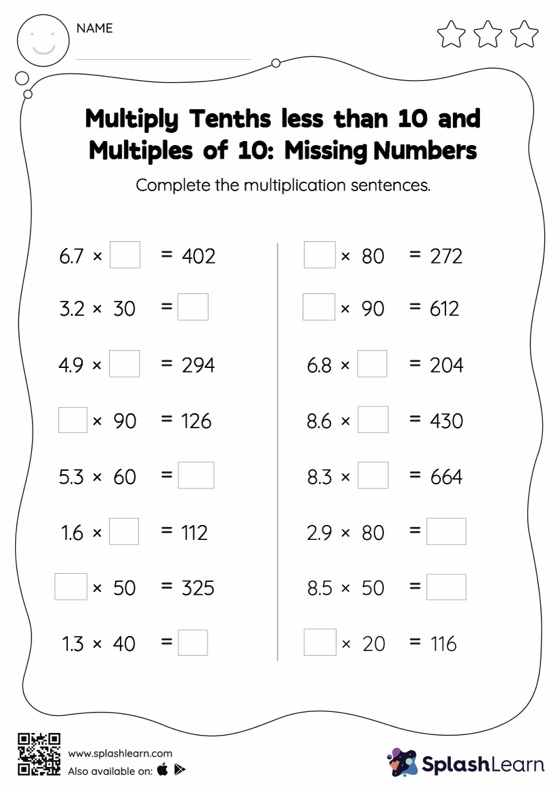# Multiply Tenths less than 10 and Multiples of 10: Missing Numbers Worksheet

Home > Multiply Tenths less than 10 and Multiples of 10: Missing NumbersChildren practice multiplying tenths less than 10 and multiples of 10 with this worksheet. Students find the missing number in decimal multiplication in multiply tenths less than 10 and multiples of 10 worksheet. To do this, they apply the understanding that when multiplying a decimal by a number that ends in zeros, the decimal point will be moved to the right by the same number of places as the multiplier's number of zeros. In each problem, the numbers are laid out in the horizontal format. Students should try to use different strategies involving composing and decomposing numbers to solve these problems. This will help them develop flexibility and fluency.